# Test: Divisibility And Remainders- 1

## 20 Questions MCQ Test Quantitative Aptitude for GMAT | Test: Divisibility And Remainders- 1

Description
Attempt Test: Divisibility And Remainders- 1 | 20 questions in 40 minutes | Mock test for GMAT preparation | Free important questions MCQ to study Quantitative Aptitude for GMAT for GMAT Exam | Download free PDF with solutions
QUESTION: 1

### X = a.bc If a, b and c denote the units, tenths and hundredths digits in the decimal representation of X above, what is the value of the product abc?      (1)  100X divided by 50 leaves a remainder 24      (2)  1000X divided by 8 leaves a remainder 0

Solution:

Steps 1 & 2: Understand Question and Draw Inferences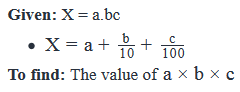Step 3: Analyze Statement 1 independently

• 100X divided by 50 leaves a remainder 24
• So, we can write: 100X=50k+24
• , where k is an integer .  .  .  (I)
• From the given expression of X, we can write: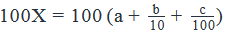• 100X=100a+10b+c .  .   .  (II)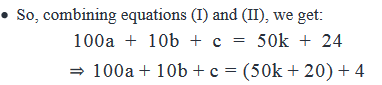• The units digit on left hand side of the equation = c
• The units digit on right hand side of the equation = 4
• This means, c = 4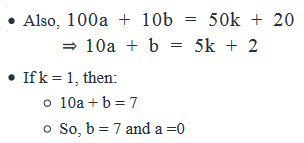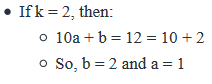• As a multiple of 5 always has its units digit equal to either 0 or 5, 5k + 2 will always have its units digit equal to 2 or 7. So, b = {2, 7}.
• However, a can have many more values.
• Thus, we only know the definite value of c
• But we do not know definite values of a and b
• Thus, we know a definite value of c
• But we do not know definite values of a and b
• So, we will not be able to find a definite value of the product a×b×c

Statement 1 is not sufficient to answer the question.

Step 4: Analyze Statement 2 independently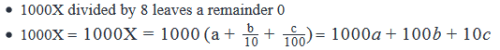• 1000a is always divisible by 8 as 1000 is divisible by 8
• 100b will be divisible by 8, only if b is even
• b= {0, 2, 4, 6, 8}
• 10c will be divisible by 8 only if c = {4, 8}
• As we do not have unique values of a, b and c we cannot calculate the unique value of product of a, b and c

Therefore, Statement 2 is clearly not sufficient

Step 5: Analyze Both Statements Together (if needed)

• From Statement 1: c = 4 and 10a+b=5k+2
• , where k is an integer
• From Statement 2: b = {0, 2, 4, 6, 8} and c = {4, 8}

So even after combining both statements, we still don’t know the definite values of a and b, and therefore cannot find a unique value of the product a×b×c

The two statements together are not sufficient to answer the question.

QUESTION: 2

### If z is a positive integer and r is the remainder when z2 + 2z + 4 is divided by 8, what is the value of r? (1) When (z-3)2 is divided by 8, the remainder is 4 (2) When 2z is divided by 8, the remainder is 2

Solution:

Steps 1 & 2: Understand Question and Draw Inferences

Given:

• Positive integer z
• z2+2z+4=8m+r,
•  where m is an integer and r is the remainder

To find: The value of r

Step 3: Analyze Statement 1 independently

• When (z-3)2 is divided by 8, the remainder is 4
• So, we can write: (z−3)2=8k+4 , where k is an integer
• That is, z2–6z+9=8k+4
• Let us try to re-express the above equation in terms of the expression in the question:
• ? z2+2z+4
• z2 – 6z +( 4+5) = 8k + 4
• So, z2–6z+4=8k−1
• Adding 8z to both sides:
• z+ 2z + 4 = 8 (k + z) - 1
• Now the expression on the left hand side of the equation is exactly the same as the expression in the question. On the right hand side, we see that the term 8(k+z) will be divisible by 8. But the remainder cannot be -1 because remainder cannot be negative. So, let’s rewrite the above equation:
• z+ 2z + 4 = 8(k + z) - 8 + 7
• z+ 2z + 4 = 8(k + z - 1) + 7
• The equation now is exactly comparable to the form 8m + r
• So, the remainder r = 7

Thus, Statement 1 alone is sufficient to answer the question.

Step 4: Analyze Statement 2 independently

• When 2z is divided by 8, the remainder is 2
• So we can write, 2z = 8n + 2, where n is some integer
• That is, z = 4n + 1
•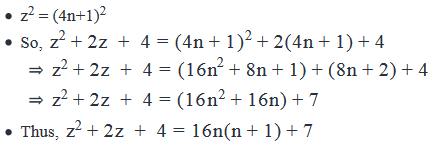• In the above equation, the term 16n(n+1)
•  will be divisible by 8
• So, the remainder when z2+2z+4  is divided by 8 will be 7

Thus, Statement 2 alone is sufficient to find a unique value of the remainder

Step 5: Analyze Both Statements Together (if needed)

Since we’ve already arrived at a unique answer in each of Steps 3 and 4, this step is not required

QUESTION: 3

### If x is an integer that lies between 390 and 400, exclusive, what is the value of x? x3 has the same units digit as x x + 2 is divisible by 9

Solution:

Steps 1 & 2: Understand Question and Draw Inferences

Given: x = {391, 392, 393 . . . 398, 399}

To find: x = ?

Step 3: Analyze Statement 1 independently

Statement 1 says that ‘x3 has the same units digit as x’

• This means that the cyclicity of units digit of x is 2. So, the units digit of x may be: {1, 4, 5, 6, 9, 0}
• We can exclude 0 from this list since x cannot be 390 or 400
• So, the possible values of x: {391, 394, 395, 396, 399}?

Since Statement 1 leads us to 5 possible values of x, it is clearly not sufficient to get us a unique answer

Step 4: Analyze Statement 2 independently

Statement 2 says that ‘x + 2 is divisible by 9’

• We know that an integer is divisible by 9 if the sum of its digits is divisible by 9
• Sum of digits of 391 = 3 + 9 + 1 = 13. So, it is not divisible by 9
• However, 391 + 5 will be divisible by 9
• Therefore, 396 will be divisible by 9.
• So, x + 2 = 396
• x = 394

• The multiple of 9 that comes before 396 is 396 – 9 = 387
• And, the multiple of 9 that comes after 396 is 396 + 9 = 405
• If we put x + 2 = 387 or 405, we get x =385 or 403. Both these values are rejected because they lie outside the given range of values for x

• Therefore, the only value of x that satisfies Statement 2 is x = 394

Statement 2 is sufficient to get us a unique answer

Step 5: Analyze Both Statements Together (if needed)

Since we’ve already got a unique answer in Step 4, this step is not required

QUESTION: 4

For a non-negative integer n, the function mod(n, d) denotes the remainder obtained when n is divided by positive integer d.  Which of the following statements must be true?

I. mod(n, d) = mod(-2n, d)

II. If mod(n, d) = 1, then mod(5n, d) = 5

III. [mod(n, d)]2  = mod (n2, d)

Solution:

Given:

• Function mod(n,d)
• n is an integer (may be zero or positive) and d is a positive integer

To find: Which of the 3 statements must be true?

Approach:

1. Since all the 3 statements contain mod(n,d), we’ll first find an expression for mod(n,d)
2. Then, we’ll evaluate the 3 statements one by one.

Working Out:

• Finding an expression for mod(n,d)
• Let mod (n,d) = r
• So, n = kd + r, where quotient k is an integer and r, being the remainder, is non-negative and less than d

• Evaluating Statement I
• mod(n, d) = mod(-2n, d)
• To evaluate Statement I, we need to find the expression of mod(-2n,d)
• mod(2n,d) will be remainder when 2n is divided by d
• 2n = 2kd + 2r
• If 2r < d, then the remainder when 2n is divided by d is 2r
• If 2r = d, then the remainder when 2n is divided by d is 0
• And if 2r > d, then the remainder when 2n is divided by d is (2r – d)
• However, as per our assumption in the Given section, mod(n, d) is r.
• So, mod(2n, d) may or may not be equal to mod(n, d)

• mod(2n, d) will be equal to mod(n,d) if r = 0. In all other cases, the 2 values will not be equal.

​​

• Thus, Statement I is not true.
• Evaluating Statement II
• If mod(n, d) = 1, then mod(5n, d) = 5
• If mod(n,d) = 1
• This means, n = kd + 1
• Therefore, 5n = 5kd + 5
• Does this mean that the mod (5n, d) = 5?
• If the divisor d is greater than 5, then yes, mod (5n, d) = 5
• But if divisor d is less than 5, then the remainder when 5n is divided by d will also be less than 5.
• So, in this case, mod (5n, d) < 5
• For example, if d = 3, then the equation 5n = 5kd + 5 will be equivalent to 15k + 5 = (15k + 3) + 2. So, the value of mod(5n,d) in this case will be 2.
• Thus, Statement II will not be true for all values of divisor d.
• Therefore, Statement II is not a must be true.

• Evaluating Statement III
• [mod(n, d)]2  = mod (n2, d)
• To evaluate Statement III, we need to find the expression of mod(n2,d)
• mod(n2,d) will be the remainder when n2 is divided by d
• Now, n2 = (kd+r)2 = k2d2 + 2kd + r2
• Does this mean that the mod(n2,d) = r2?
• If the divisor d is greater than r2, then the answer is Yes
• But, if divisor d is less than or equal to r2, then the answer is No.
• For example, let’s consider the case where d = 25 and r = 5, that is, n = 25k + 5
• So, n2 = 252k2 + 250k + 25 = 25(25k2 + 10k + 1). So, when n2 is divided by divisor d = 25, the remainder is 0.

• Therefore, Statement III is not true for all values of d.
• So, it is not a must be true statement.

• Thus, we see that none of the 3 statements is a must be true statement.

Looking at the answer choices, we see that the correct answer is Option E

QUESTION: 5

When a class of n students is divided into groups of 6 students each, 2 students are left without a group. When the class is divided into groups of 8 students each, 4 students are left without a group. What is the smallest number of students that can be added to or removed from the class so that the resulting number of students can be equally divided into groups of 12 students each?

Solution:

Given:

• n = 6k + 2 = 8p + 4, where k and p are non-negative integers (if k or p were negative, then n would be negative too and that’s not possible since the number of students cannot be negative)

To find: The smallest number that can be added to or subtracted from n to make the resulting number divisible by 12

Approach:

1. Let the number to be added or subtracted from n be x. This means,

• Either (students are added) n + x = 12j, where j is a positive integer
• This means, n = 12j - x
• Or (students are removed) n – x = 12m, where m is a positive integer
• This means, n = 12m + x

2. The expressions n = 12j – x and n = 12m + x make us realize that in order to answer this question, we need to know the remainder when n is divided by 12

• Say the remainder is 3 (That is, n = 12m + 3). In this case, the smallest change to make the number of students divisible by 12 is to remove 3 students from the class.
• Same will be the case for any value of remainder less than or equal to 6

• Say the remainder is 10 (That is, n = 12m + 10). In this case, the smallest change to make the number of students divisible by 12 is to add 2 students to the class
• Same will be the case for any value of remainder greater than 6

3. Let the remainder that n leaves when divided by 12 be r. So, our Goal expression is: n = 12q + r, where quotient q is an integer and 0 ≤ r < 12

4. We’ll use the given information about n to drive towards our Goal expression

Working Out:

• n = 6k + 2 = 8p + 4
• LCM (6, 8) = 24
• This means, n is a number of the form 24z + r’, where 0 ≤ r’ < 24
• When 24z + r’ is divided by 6, the remainder is 2
• In this expression, the term 24z is divisible by 6
• So, possible values of r’ that can lead to remainder 2 = {2, 8, 14, 20}

• When 24z + r’ is divided by 8, the remainder is 4
• In this expression, the term 24z is divisible by 8
• So, possible values of r’ that can lead to remainder 4 = {4, 12, 20}

• The only value of r’ that satisfies both these conditions = {20}
• So, r’ = 20

• Therefore, n = 24z +20

• Let’s now rearrange the above equation a little bit to make it comparable to our GOAL expression: n = {24z + 12} + 8
• Now this form is exactly comparable to our Goal Expression
• By comparison, we see that Remainder = 8
• Since remainder is greater than 6, the smallest change to make the number of students divisible by 12 is to add 4 students.

Looking at the answer choices, we see that the correct answer is Option B

QUESTION: 6

If x is a positive integer and f(x) = x – x2 – x3 + x4 + x5 – x6 –  x7 + x8 , then is f(x) divisible by 96?

(1) x – x2 – x3 is divisible by 32

(2) x has no prime factors other than 2

Solution:

Steps 1 & 2: Understand Question and Draw Inferences

Given:

• Integer x > 0
• f(x) = x – x2 – x3 + x4 + x5 – x6 –  x7 + x8

= x(1-x) – x3(1-x) +x5(1-x) – x7(1-x)

=(x – x3 + x5 – x7)(1-x)

=[x(1-x2) +x5(1-x2)](1-x)

=x(1+x4)(1-x)(1+x)(1-x)

• Rearranging the terms in ascending order: (x-1)2(x)(x+1)(x4+1)

To find:

• Is (x-1)2(x)(x+1)(x4+1) divisible by 96?
• 96 = 3*25
• The answer to the question is YES only if:
• f(x) is divisible by 3

The expression for f(x), that is (x-1)2(x)(x+1)(x4+1), contains the product of 3 consecutive integers ( x-1, x and x+1 ).Therefore, f(x) is definitely divisible by 3 (because in any set of 3 consecutive integers, one will definitely be a multiple of 3) ​

• And f(x) is divisible by 25 (that is, 32)

Let’s evaluate the conditions in which f(x) will be divisible by 25

The divisibility of f(x) by powers of 2 will be impacted by the even-odd nature of x

Case 1: x is even

• Then x–1,x+1 and x4+1
• are all odd
• So, (x−12)(x)(x+1)x4+1)=(Odd)∗ Even∗Odd∗Odd
• So, f(x) will be divisible by 32 only if x is divisible by 32.

Case 2: x is odd

• ​​Then x–1,x+1andx4+1
• are all even
• So, (x−12)(x)(x+1)x4+1)=(Even)2∗Odd∗Even∗Even
• Since f(x) contains 4 even terms, it will be definitely be divisible by 24
• Also, since two of these even terms are consecutive even numbers (x - 1 and x + 1), one of them will also be divisible by 4.
• Therefore, f(x) will definitely be divisible by 25 = 32

• So, the answer to the question will be YES only if
1. Either x is even and divisible by 32
2. Or x is odd

Step 3: Analyze Statement 1 independently

• x–x2–x3
•  is divisible by 32
• x(1–x–x2)
•  is divisible by 32
• x is either Even or Odd
• Case 1: x is even
• ​1−x−x2=Odd−Even−Even=Odd
• Since(1–x–x2)  is odd, it cannot be divisible by 32
• So, the only way Statement 1 can hold true is if x is divisible by 32
• Since x is even and divisible by 32, the answer to the question is YES in this case

Case 2: x is odd

• 1−x−x2=Odd−Odd−Odd=Odd
• Since(1–x–x2)  is odd, it cannot be divisible by 32
• And, since x is odd, it too cannot be divisible by even number 32
• Thus, the product x(1–x–x2)  cannot be divisible by 32 if x is odd
• Since Statement 1 tells us that x(1–x–x2)  is in fact divisible by 32, the natural conclusion to draw is that x cannot be odd.
• Thus, from Statement 1, we deduce that x is even and divisible by 32
• So, the answer to the question is YES

Therefore, Statement 1 is sufficient.

Step 4: Analyze Statement 2 independently

• x has no prime factors other than 2
• x is even
• But is x divisible by 32? Maybe, maybe not. For example, x = 4 and 64 both satisfy Statement 2, but 64 is divisible by 32 whereas 4 is not.

Thus, Statement 2 alone is not sufficient to arrive at a definite answer to the question.

Step 5: Analyze Both Statements Together (if needed)

Since we’ve already arrived at a unique answer in Step 3, this step is not required

QUESTION: 7

Set A = {20, P, 80}

Set B = {1, 3, 5, 23}

When the mean of the Set A is divided by the median of the Set B, the result is 15.25. What is the remainder when P4 is divided by the mean of the Set B?

Solution:

Given: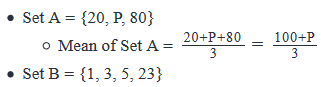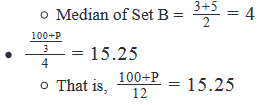To find: The remainder when P4 is divided by the mean of Set B

Approach:

1. To answer the question, we need to know the values of P and the mean of Set B
• We can use the relation inferred above,((100+P)/12)=15.25 , to determine the value of P
• Since we know all the elements of Set B, we can easily find the mean of Set B

Working Out: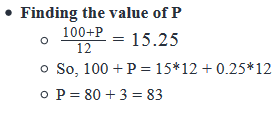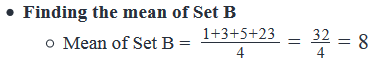• Finding the required remainder
• P4 = 834 = (80+3)4
• From Binomial Theorem, we know that all terms in the expansion of the above expression, except the last term, will contain 80 and hence will be divisible by 8.
• Last term = 34 = 81.
• So, P4 is an expression of the form 8k + 81 = 8(k+10) + 1
• So, the remainder when P4 is divided by 8 will be 1

Looking at the answer choices, we see that the correct answer is Option A

(Note: To get more practice on Binomial Theorem, you may look at the Divisibility and Remainders Diagnostic Test – 2 questions in that test make use of the Binomial Theorem and their solutions contain a detailed explanation of Binomial Theorem. If you are a Quant Live Prep student, you may also look at the Divisibility questions in Number Properties 2 Live Session)

QUESTION: 8

The arithmetic mean (average) M of 4 terms is an integer. When M is divided by 16, the remainder is 10. If each of the terms is increased by 100%, what is the remainder when the new mean is divided by 16?

Solution:

Given: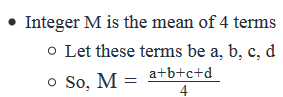• M = 16q + 10, where q is an integer
• Each term is increased by 100%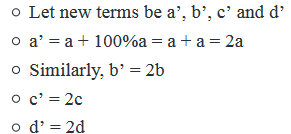To find: The remainder when the new mean is divided by 16

Approach:

1. Let the new mean be M’ and the required remainder be r?
• To answer the question, we need to express M’ in the form 16k + r, where k is an integer and 0 ≤ r < 16?
•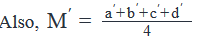2. Since we’re given the relation M = 16q + 10, we’ll express M in terms of M’ and thus get an expression for M’ in the form 16k + r

Working Out: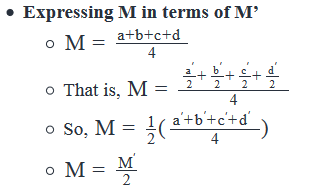• Finding the required remainder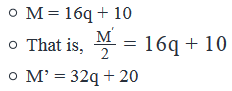• This expression can be rearranged as: M’ = (32q+16) + 4
• So, the form M’ = (32q+16) + 4 is comparable to the form 16k + r
• And 4 is less than the divisor 16
• In this form, we see that the term 32q+16 is divisible by 16. So, it is of the form 16k
• Therefore, the remainder when M’ is divided by 16 is 4

Looking at the answer choices, we see that the correct answer is Option B

QUESTION: 9

The students of a class are to be arranged in rows, starting from number 1,  such that a row can have a maximum of x students, where x is a number to be determined that is greater than 1. If the minimum number of rows required to accommodate all the students is 10,  what is the number of students in the class?

(1) If 3 students are shifted from row number 9 to row number 10, both the rows would have an equal number of students.

(2) Had there been 1 student less in the class, the minimum number of rows required would have been 9.

Solution:

Steps 1 & 2: Understand Question and Draw Inferences

Given:

• Maximum number of students in a row = x
• Minimum number of rows required to accommodate all the students = 10

Since we are talking about minimum number of rows
Two cases are possible:

1. Number of students when all the 10 rows consist of x students each = 10x
2. Number of students when the last row (10th row) is not completely occupied = 9x + z, where z is the number of students in the last row.

We need to find the values of x and z.

Step 3: Analyze Statement 1 independently

1. If 3 students are shifted from row number 9 to row number 10, both the rows would have an equal number of students.

Statement I conveys that a shifting of students is required to achieve an equal number of students in Rows 9 and 10. This means that the number of students in these two rows initially is not equally. So, what we know for sure now is that Case a is rejected. It is Case-b that is applicable.

As per Case b, Number of students in 9th row = x

Number of students in 10th row = z

As per Statement 1, x -3 = z + 3

So, x = z +6.

2 unknowns and 1 equation. We cannot find unique values of x and z. Insufficient to answer the question

Step 4: Analyze Statement 2 independently

(2) Had there been 1 student less in the class, the minimum number of rows required would have been 9.

This means, there is only 1 student in the last row. Case-b is applicable.

z = 1. However, it does not tell us about the value of x. Insufficient to answer

Step 5: Analyze Both Statements Together (if needed)

St- 1 gives us relation between x and z and St-2 gives us the value of z. Thus, we can find a unique value of x.

QUESTION: 10

A function D(a, 10b + c) is defined as the remainder when the sum a0 + a1….a10b + c is divided by c, where a, b and c are single-digit positive integers.

What is the value of D(y, 10x + z) where x, y and z are single-digit positive integers such that x < y < z ,  x and z are perfect squares and the difference between the sum and the product of the prime factors of y is 1?

Solution:

Given

• D(a, 10b + c) =remainder when (a0 + a1….a10b + c ) is divided by c
• a, b and c are single-digit integers > 0
• x, y , z are single digit integers > 0
• x < y < z
• x = p2 and z = q2, where p, q are integers
•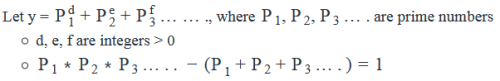• If y has only one prime factor then, then the difference between the product and the sum of prime factors would be 0
• So, we can say that y has more than 1 prime factor

To Find: Value of D(y, 10x + z)

• That is, the value of the remainder when y0+y1…..y10x+z  is divided by z
• Approach

• For finding the remainder, we need to find the value of x, y and z
• Finding value of y
1. We have inferred that y has more than one 1 prime factor.
2. Also, we will consider the possible combinations of 2 or more prime factors keeping in the mind the constraint that y lies between 2 distinct single digit perfect squares
1. There are 3 single digit perfect squares = {1, 4, 9}
• Finding values of x and z
1. We are given that x =p2 and z =q2. Also, as x and z are integers < 10, the perfect squares less than 10 can be 1, 4 or 9.
2. We will apply the constraints given on x and z to determine their values.
•

Working Out

• Finding value of y
1. We have inferred that y has more than one prime factor.
2. Now, the smallest prime factors are {2, 3, 5, 7…}
3. We will see which combinations of the above prime factors results in a value of y < 10
4. From the above set, we can see that there is only one possible combination for which the value of y < 10, i.e. y = 2* 3 = 6
5. So, the product of prime factors of y = 6 and the sum of prime factors of y = 5.
1. Therefore the difference between the product and the sum of prime factors of y = 1
6. Hence y = 6

• Finding values of x and z
1. Perfect squares less than 10 = {1, 4, 9}.  We are not assuming 0 here, as we are given that x, y and z are positive.
2. As x < z, the possible values of (x, z) = (1, 4) , (1, 9) or (4, 9)
3. Since y = 6 and x < y < z, (x, z) ≠ (1, 4)
4. So, (x, z) = (1, 9) or (4, 9)

3. Let’s find the value of  D(y, 10x + z) for both the cases of values of (x, z)

a. Case-I: y = 6 and (x, z) = (1, 9)​​​

• 60+61……619  is to be divided by 9 = 32
• Since 6=2∗3,62=22∗32
• So, all the powers of 6 greater than 1, will be divisible by 9
• Therefore D(6, 10 +9) = Remainder when 60+61(=1+6=7)
•   is divided by 9 = 7
• So, D(6,10 + 9) =

b. Case-II: y = 6 and (x, z) = (4, 9)

• 60+61……649  is to be divided by 9 = 32
• Since 6=2∗3,62=22∗32
• So, all the powers of 6 greater than 1, will be divisible by 9
• Therefore D(6, 40 +9) = Remainder when 60+61
• (= 1 + 6 + 7) is divided by 9 = 7
• So, D(6, 10 + 9) = 7
• 4. We see that in both the cases, the value of D(x, 10y+z) = 7.

QUESTION: 11

The arithmetic mean (average) of a set of 5 numbers is an integer A. Upon being divided by 180, A leaves a remainder of 30. If two of the numbers in the set are decreased by 120 and 180 units respectively, which of the following statements must be true about the new arithmetic mean B of the set?

I. The remainder that B leaves upon division by 180 is less than the remainder that A leaves upon division by 180

II. When B is divided by 60, the remainder is 30

III. The value of the decimal digits is greater in the number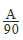than in the number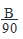Solution:
• Let the set of 5 numbers be {p, q, r, s, t}
• Arithmetic Mean of this set = A
•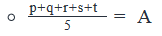• A is an integer
• A = 180q + 30, where quotient q is an integer
• When 2 numbers in the set are decreased by 120 and 180, the new Arithmetic Mean is B

To find: Which of the 3 statements is/are true?

Approach:

• Looking at the statements, we realize that to answer the question, we need to find an equation for B (to find the remainder that B leaves with certain divisors) and also a relation between A and B
• We’ll use the given information to find these 2 information points
• Since we are given the decrease in 2 numbers of the set, we can find the total decrease in the sum of the 5 numbers (with respect to the original sum of the 5 numbers). By expressing the original sum of the 5 numbers and the decreased sum in terms of A and B respectively, we can find a relation between A and B
• Since we know an equation for A, we can use the relation between A and B to find an equation for B.
• Then, we’ll evaluate the 3 statements one by one.
• Working Out:

• Finding a relation between A and B
• When 2 numbers in the set are decreased by 120 and 180, the new Arithmetic Mean is B
• 120 + 180 = 300
• So, when the sum p  +q + r + s + t is decreased by 300, the new mean is B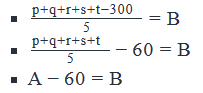Thus, B = A – 60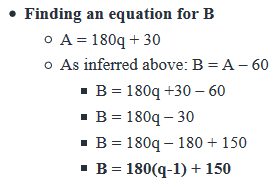• Evaluating Statement I
• The remainder that B leaves upon division by 180 is less than the remainder that A leaves upon division by 180
• The remainder that A leaves with 180 is 30
• From the equation B = 180(q-1) + 150, it is clear that the remainder that B leaves with 180 is 150
• Since 150 is greater than 30, Statement I is not true.

• Evaluating Statement II
• When B is divided by 60, the remainder is 30
• Rearranging the equation B = 180(q-1) + 150:
• B = 180(q-1) + 120 +30
• B = {180(q-1) + 120} + 30
• From the above form of the equation, it’s clear that when B is divided by 60, the term {180(q-1) + 120} will be divisible by 6. The leftover term, 30, is positive and is less than the divisor 60. So, it passes both the tests for being a remainder.
• Therefore, when B is divided by 60, the remainder is 30
• Statement II is true.

• Evaluating Statement III
• The value of the decimal digits is greater in the numberthan in the number• A = 180q + 30
• So, when A is divided by 90, the term 180q is divisible by 90. The decimal digits come from the part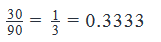• B = 180(q-1) + 150 = {180(q-1) + 90}  + 60
• So, when B is divided by 90, the term {180(q-1) + 90} is divisible by 90. The decimal digits come from the part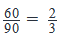• Therefore, the value of the decimal digits is greater in• So, Statement III is not true.

• Thus, we see that only Statement II is true.

Looking at the answer choices, we see that the correct answer is Option B

QUESTION: 12

If x is a positive integer, what is the remainder when 15x + x15 is divided by 4?

(1) The sum of the product of x and z and the sum of x and z is even, where z is a positive integer.

(2) y when divided by x is equal to 13.45, where y is a positive integer

Solution:

Steps 1 & 2: Understand Question and Draw Inferences

Given:

• Integer x > 0

To Find: The remainder when 15x + x15 is divided by 4

• We will be using the binomial theorem to determine the divisibility of the expression
• Analyzing first both the terms independently:
• 15x = (16 - 1)x
• When expanded, each term of (16 - 1)x will consist of 16 except the last term
•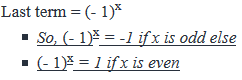• x15
• If x is even, x15 will be divisible by 4
• If x is odd, x15 = (2n + 1)15
• When expanded, each term of (2n + 1)15  will consist of at-least (2n)2= 4n2 except the last 2 terms
• The last two terms will be 2n + 1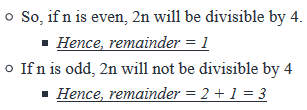Combining the analysis of both the terms: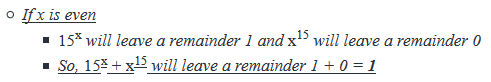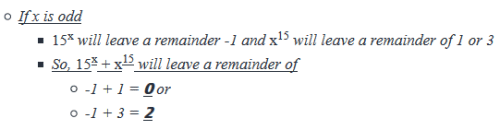• Sum and product of two numbers is even when
• Both the numbers are even.
• So, x is even
• When x is even, remainder = 1

Statement-1 is sufficient to answer the question

Step 4: Analyze Statement 2 independently

(2) y when divided by x is equal to 13.45, where y is a positive integer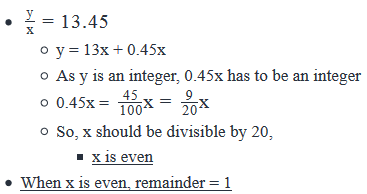Statement-2 is sufficient to answer the question

Step 5: Analyze Both Statements Together (if needed)

As we have a unique answer from steps-3 and 4, we do not need this step

QUESTION: 13

If x is a prime number, which of the following statements must be true?

1. x3 – x is divisible by 3
2. x2 – 1 is divisible by 4
3. x2 + x is divisible by 6
Solution:

Given:  Prime number x

To find: Which of the statements must be true?

Approach:

1. A statement is must-be-true if it holds true for all possible values of x. So, we will evaluate the 3 statements one by one, keeping in mind the constraint that x is a prime number.

Working Out:

• Evaluating Statement I
• As per Statement I, x3 – x is divisible by 3
• Simplifying the expression x3 – x:
• x3 – x = x(x2 – 1) = x(x+1)(x-1)
• Rearranging the terms: (x+1)*x*(x-1)
• This is the product of 3 consecutive integers
• Therefore, it will definitely be divisible by 3
• So, Statement I is a must be true statement.

• Evaluating Statement II
• As per Statement II, x2 – 1 is divisible by 4
• Simplifying the expression x2 – 1:
• x2 – 1 = (x + 1)(x – 1)
• If x is odd, then both x + 1 and x – 1 will be even. So, the product of these 2 even terms will be divisible by 4
• However, x may also be 2 (the only even prime number). In that case, the terms x  +1 and x – 1 are both odd. So, their product will be odd as well, and so, will not be divisible by 4
• Thus, Statement II does not hold true for one possible value of x
• Therefore, it is not a must be true statement

• Evaluating Statement III
• As per Statement III, x2 + x is divisible by 6
• 6 = 2*3; So, a number will be divisible by 6 if it is divisible by both 2 and 3
• Simplifying the expression x2 +x:
• x2 + x = x(x+1)
• The expression x(x+1) is the product of 2 consecutive integers. So, it will definitely be even
• So, this expression is divisible by 2
• However, the product of 2 consecutive integers may or may not be divisible by 3:
• If x = 2, then x  +1  = 3; So, x(x+1) will be divisible by 3
• If x = 3, then of course x(x+1) will be divisible by 3
• If x = 5, then x + 1 = 6; So, x(x+1) will be divisible by 3
• If x = 7, then x + 1 = 8; So, x(x+1) is NOT divisible by 3
• Thus, we see that for some possible values of x, the given expression will be divisible by 3 (and hence by 6) and for others, it will not be.
•  So, Statement III is not a must be true statement

• Thus, we’ve evaluated that only Statement I is a must be true statement

So, the correct answer is Option A

QUESTION: 14

The expression 2a2 +2b2 can be written in the form of 18x + y, where a, b, x and y are non-negative integers and y < 18. Is |y+3| = 3?

(1) The difference between a and b can be expressed as an even multiple of 3.

(2) b when divided by 3 is an integer.

Solution:

Steps 1 & 2: Understand Question and Draw Inferences

• a, b, x and y are integers ≥ 0 and y < 18
• 2a2+2b2=18x+y
• Since both the terms on the left hand side of the above equation are even, their sum will be even as well. So, the left hand side of the equation is even
• Therefore, the right hand side of the equation will be even as well
• So, the sum of even term 18x and of y will be even
• This means, y must be even.

To Find: If |y+3| = 3?

• Let’s first understand what are the values of y for which this equation will hold true.
• So, let’s first solve for the values of y that satisfy this equation and then, using Statements 1 and 2, we will figure out if y does indeed have those values.
• For the equation |y + 3| = 3, two cases are possible:
• If y+3 ≥ 0
• Then y+3 = 3, i.e. y = 0
• If y +3 < 0
• -y  -3 = 3, i.e. y = -6. However as y ≥ 0, this case is not possible.
• So, y = 0 is the only possible value of y, under the given constraints, for which |y + 3| = 3
• So, the answer to the question will be YES only if y = 0.
• Now, when y = 0, then the equation 2a2 + 2b2 = 18x + y becomes:
• Hence, we can write 2a2+2b2=18x
•
• That is, a2 + b2 = 9x
• That is, a2 + b2 is divisible by 9
• So, if we find that a2 + b2 is divisible by 9, then that will mean that y = 0, which in turn means that the answer to the asked question is YES.

Step 3: Analyze Statement 1 independently

1. The difference between a and b can be expressed as an even multiple of 3.
• a – b = 3*2k, where k is an integer
• Two cases arise
• If (a-b) ≥0, |a-b| = (a-b)
• So, we have ,
• a  = b + 6k
• Substituting a = b +6k in a2+b2, have
• (b+6k)2+b2=2b2+36k2+12bk
• 36k2 is divisible by 9, but we do not know if 2b2 + 12bk is divisible by 9.
• If (a-b) < 0, |a-b| = (b-a)
• So, we have
• b = a + 6k
• Substituting b= a +6k in a2 + b2, we have
• a2+(a+6k)2=2a2+36k2+12ak
• 36k2 is divisible by 9, but we do not know if 2a2 + 12ak is divisible by 9.

Step 4: Analyze Statement 2 independently

2.   b when divided by 3 is an integer.

• b = 3p, where p is an integer > 0
• However the statement does not tell us anything about a.

Step 5: Analyze Both Statements Together (if needed)

1. From statement-1,
1. a2+b2=2b2+36k2+12bk or
2. a2+b2=2a2+36k2+12ak
2. From statement-2, b = 3p
• Substituting (2) in (1. a), we have
•  a2+b2=18p2+36k2+36pk
• All the terms 18p2+36k2+36pk  are divisible by 9, hence we can say that a2+b2=9x
• For (1.b), we know that
• b = a + 6k, i.e. a = b – 6k = 3p – 6k
• Substituting a = 3p – 6k and b = 3p in a2 + b2, we have
• a2+b2=(3p−6k)2+9p2=18p2+36k2−36pk
• All the terms 18p2+36k2−36pk are divisible by 9, hence we can say that a2+b2=9x

QUESTION: 15

If k and m are positive integers, what is the remainder when k is divided by m +1?

(1) k-m when divided by m+1 leaves a remainder 1

(2) k/3 and k/2 when divided by m+1 leave a remainder equal to 4 and 3 respectively.

Solution:

Steps 1 & 2: Understand Question and Draw Inferences

• k, m are integers > 0

To Find: remainder when k is divided by m +1?

• Value of r in k = (m+1)x + r,
• x is an integer ≥ 0
• where 0 ≤ r < (m+1)

Step 3: Analyze Statement 1 independently

1. k-m when divided by m+1 leaves a remainder 1
• k – m = (m+1)y + 1, where y is an integer ≥ 0
• k = (m+1)y + m +1
• k = (m+1)* ( y +1)
• Thus, k is divisible by m +1 and hence it leaves a remainder 0
• r = 0

Step 4: Analyze Statement 2 independently

1. k/3   and k/2  when divided by m+1 leave a remainder equal to 4 and 3 respectively.
• k/3=(m+1)a+4 , where a is an integer ≥ 0
• k=(m+1)∗3a+12…………..(1)
• It seems from above that 12 is the remainder when k is divided by (m+1)
• However, we know that remainder(i.e. 12) should always be less than the  divisor (i.e. (m+1))
• Since, we do not know if 12 < m +1. So, we cannot find a unique value of r from it.
• k/2=(m+1)b+3 , where b is an integer ≥ 0
• k=(m+1)∗2b+6…………….(2)
• As discussed in the above case, here also we do not know if 6 < m +1. So, we cannot find a unique value of r from it.
• Since we need to express k in terms of (m+1), let’s try to eliminate the constants using equations (1) and (2)
• Carrying out the operation, (2) * 2 – (1), we get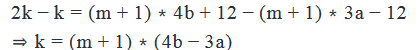• Thus k is divisible by (m+1) and hence leaves a remainder 0
• So, r = 0

Step 5: Analyze Both Statements Together (if needed)

As we have a unique answer from steps 3 and 4, this step is not required.

QUESTION: 16

If n is a perfect square of a positive integer and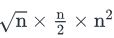is an integer, then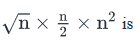Solution:

Given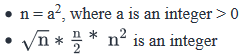• As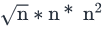is divisible by 2, that means n must be even. So, n must have 2 as one of its prime factors

To Find: To check the divisibility of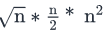in the given options

Approach

1. Since n has 2 as one of its prime factors, we will find out the minimum possible power of 2 in the expression2. Based on this deduction and the assumptions given in the options, we would check the options for their correctness.

Working Out

• Since n is a perfect square, the power of every prime factor in n will be even. We’ve already established that 2 is a prime factor of n. So, n will at-least have 2in it.
1. Therefore √n will have at-least 2in it and n2  will at-least have 24 in it.
2. Hence, the expressionwill definitely have 2 = 64 in it. Let’s check the options now.

2. Evaluating the given options:

• Divisible by 3 → We do not know if n has 3 as one of its prime factors. So, the expression may or may not be divisible by 3.
• Divisible by 128 → The expression is definitely divisible by 64. It may or may not be divisible by 128.
• Divisible by 272 if n is a multiple of 3 → If n has 3 as one of its prime factors,will definitely have 37 in it. As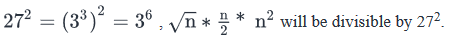• Now, we do not need to analyse further options as we have our answer in option C. However, we are doing it for the sake of completeness of the solution.
• Divisible by 107 if n is a multiple of 5→ If n is a multiple of 5,will definitely have 57 in it. We also know thathas 26 in it. So, the expression must have 106 * 5  in it. So, it may or may not be divisible by 107.

QUESTION: 17

If x and y are positive integers such that x/y=5.4, which of the following could be the value of y?

Solution:

Given: Integers x, y > 0

x/y=5.4,

To find: The possible value of y

Approach:

1. To find the possible value of y, we will make use of the constraints on x and y:
1. Constraint 1: xy=5.4,
1. From this constraint, we will be able to express x in terms of y
2. Constraint 2: y is a positive integer, and so is x
1. From the expression obtained for x in terms of y, we will evaluate for what values of y will x be an integer. These will be the possible values of y

Working Out: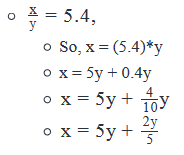We are given that x is an integer.

• This means the sum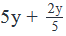is an integer.
• In this sum, the term 5y is definitely an integer (since we know that y is an integer).
• So, the other term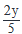must be an integer as well
• This will happen only if y is a multiple of 5
• Thus, we conclude that only those values of y will satisfy both Constraints 1 and 2 in which y is a multiple of 5
• Looking at the answer choices, we see that the only value that is a multiple of y is Option D, 15.

QUESTION: 18

If x is a positive integer such that x - 1 and x + 6 are not divisible by 3, which of the following must be divisible by 3?

Solution:

Given: Integer x > 0

• x - 1 is not divisible by 3
• x + 6 is not divisible by 3

To find: Which of the given 5 terms is divisible by 3?

Approach:

1.  We know that when an integer is divided by 3, there are only 3 possible remainders: {0, 1, 2}
• Therefore, every integer can be expressed in one of the 3 forms: 3k, 3k + 1, 3k + 2, where quotient k is an integer
2. Using the information that x - 1 and x + 6 are not divisible by 3, we will be able to rule out one or more forms of the 3 forms discussed in Step 1. Thus, we will have a clearer idea of what form does integer x belong to.
3. Then, we will use this information to evaluate the 5 options

Working Out:

• x - 1 is not divisible by 3
• This means, x - 1 is not of the form 3k
• So, x is not of the form 3k + 1

• x + 6 is not divisible by 3
• Note that in the sum x + 6, the term 6 is definitely divisible by 3
• Therefore, it is x that is not divisible by 3
• So, x is not of the form 3k

• As discussed in the Approach, every integer can be expressed in one of the 3 forms: 3k, 3k + 1, 3k + 2, where quotient k is an integer
• We’ve worked out that x is not of the form 3k or 3k + 1
• Therefore, x must be of the form 3k + 2

• Since x = 3k + 2, x + 1 ( = 3k + 3) will be divisible by 3. Then, (x + 1) + 3, that is x + 4 will be divisible. After this, (x + 4) + 3 = x + 7 will be divisible by 3 and so on.
• Moving on the left hand side of x on the number line, we see that x -2 ( = 3k) will be divisible by 3. Then, (x – 2) – 3 = x – 5 will be divisible by 3. After that, (x – 5) – 3 = x – 8 will be divisible by 3 and so on.
• So, to sum up, the pattern of the numbers that will be divisible by x is as under:
• . . . (x-8), (x-5), (x-2), (x+1), (x + 4), (x+7), (x + 10) . . .

Looking at the answer choices, we see that (x + 7) is one of the answer choices. So that is the correct answer – Option D.

QUESTION: 19

If T is a prime number, what is the remainder when T is divided by 3?

(1) (T – 13)3 is divisible by 48

(2) 4T – 3 leaves a remainder 1 when divided by 18

Solution:

Steps 1 & 2: Understand Question and Draw Inferences

Given: Prime Number T

To find: Remainder when T is divided by 3

• To answer this, we need to express T in the form 3k + r where k is an integer and r = {0, 1, 2}
• Please note that r = 0, only in the case when T = 3. For all the other values of T, T will not be divisible by 3.

Step 3: Analyze Statement 1 independently

• (T – 13)3 is divisible by 48
• 48 = 24 * 3
• Since T is an integer, T – 13 will be an integer as well. Let T – 13 = N
• So we can write: N3 is divisible by 24 and by 3
• Since N3 is divisible by 24, N must be divisible by 22
• Explanatory note:
1. Since N3 is divisible by an even number (24), this means that 2 is definitely a prime factor of N3, and hence, of N
2. So, we can write: N = 2a * P2b *P3c * .  .  . , where P2, P­3 etc. are the other prime factors of N
3. And, N3 = 23a * P23b *P33c * .  .  . ,
4. Since N3 is divisible by 24, this means: 23a≥24
5. Comparing the powers of 2, we can write: 3a≥4
6. That is, a≥4/3
7. Since N is an integer, the power of 2 cannot be a fraction. So, a must be an integer as well
8. What is the smallest integer that is greater than 4/3 ? It is 2
9. So, amin = 2
10. Thus, N must be divisible by 22

• Thus, N is divisible by 22 (that is, 4) and by 3
• Least common multiple of 4 and 3 is 12
• Thus, N is divisible by 12

• So T – 13 is divisible by 12
• T – 13 is of the form 12q, where q is some integer
• T = 12q + 13
• T = 12q + 12 + 1
• T = 12(q + 1) + 1
• In the above expression, the term 12(q+1) is divisible by 3. Let the quotient when this term is divided by 3 be k
• So, we can write: T = 3k + 1
• Therefore, Remainder r = 1

Statement 1 alone is sufficient to answer the question.

Step 4: Analyze Statement 2 independently

• 4T – 3 leaves a remainder 1 when divided by 18
• So, we can write: 4T – 3 = 18m + 1, where quotient m is some integer
• 4T - 4 = 18m
• 2T - 2 = 9m
•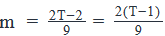• Since m is an integer, the product 2(T - 1) must be divisible by 9
• In this product, 2 is clearly not divisible by 9
• So, T – 1 must be divisible by 9
•   So, we can write: T – 1 =9z, where z is some integer
• Thus T = 9z + 1
• In the above expression, the term 9z is divisible by 3
• So, the remainder obtained when T is divided by 3 is 1
• Thus, Statement 2 alone is also sufficient to obtain a unique answer

Step 5: Analyze Both Statements Together (if needed)

Since we’ve arrived at a unique answer in each of Steps 3 and 4, this step is not required

QUESTION: 20

Is the square of positive integer Z divisible by 9?

(1)  The sum of the digits of Z3 is divisible by 9

(2)  3Z4 + 16 leaves a remainder of 7 when divided by 9

Solution:

Steps 1 & 2: Understand Question and Draw Inferences

Given: Positive integer Z

To find:

• Is Z2 divisible by 9?
• 9 = 32
• So, Z2 will be divisible by 32 if
• Z is divisible by 3

Step 3: Analyze Statement 1 independently

• The sum of the digits of Z3 is divisible by 9
• This means, Z3 is divisible by 9
• 9 = 32
• So, 3 is a prime factor of Z3
• Therefore, 3 is a prime factor of Z as well

Hence, Z is indeed divisible by 3

Statement 1 is sufficient to answer the question.

Step 4: Analyze Statement 2 independently

• 3Z4 + 16 leaves a remainder of 7 when divided by 9
• So, we can write: 3Z4 + 16 = 9k + 7, where k is some integer
• 3Z4 = 9k + (7 – 16) = 9k – 9 = 9(k-1)
• This simplifies to: Z4  = 3(k-1)
• Z4 is divisible by 3
• In other words, 3 is a prime factor of Z4
• Therefore 3 is a prime factor of Z as well.

So, Z is indeed divisible by 3

Statement 2 alone is sufficient to answer the question.

Step 5: Analyze Both Statements Together (if needed)

Since we’ve arrived at a unique answer in each of Steps 3 and 4, this step is not requiredUse Code STAYHOME200 and get INR 200 additional OFF Use Coupon Code